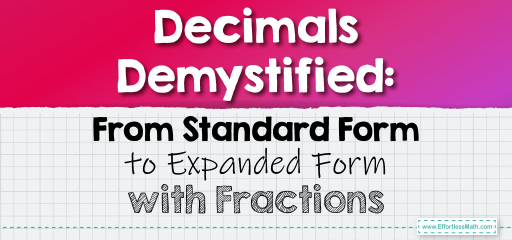# Decimals Demystified: From Standard Form to Expanded Form with Fractions

Decimals can be expressed in various ways, and when we talk about the expanded form, it's not just limited to decimals. We can also use fractions to represent the values after the decimal point. This approach offers a deeper understanding of the value each digit represents. Let's dive into the conversion process!## Converting Decimals Between Standard and Expanded Form Using Fractions

### Example 1:

Standard Form: $$4.25$$

Expanded Form with Fractions:

– The digit $$4$$ is in the ones place: $$4$$

– The digit $$2$$ is in the tenths place, which can be represented as $$\frac{2}{10}$$ or $$1/5$$.

– The digit $$5$$ is in the hundredths place, which can be represented as $$\frac{5}{100}$$ or $$1/20$$.

Combine these values: $$4 + \frac{1}{5} + \frac{1}{20}$$

The expanded form of $$4.25$$ using fractions is $$4 + \frac{1}{5} + \frac{1}{20}$$.

The Absolute Best Book for 5th Grade Students

### Example 2:

Standard Form:** $$3.08$$

**Expanded Form with Fractions:**

– The digit $$3$$ is in the ones place: $$3$$

– The digit $$0$$ is in the tenths place, which is $$0$$.

– The digit $$8$$ is in the hundredths place, which can be represented as $$\frac{8}{100}$$ or $$2/25$$.

Combine these values: $$3 + \frac{2}{25}$$

The expanded form of $$3.08$$ using fractions is $$3 + \frac{2}{25}$$.

Remember using fractions in the expanded form of decimals offers a unique perspective on the value each digit represents. It bridges the gap between decimals and fractions, two essential concepts in mathematics. With practice, you’ll find it easy to switch between these representations seamlessly!

### Practice Questions:

1. Convert the decimal $$1.75$$ into its expanded form using fractions.

2. Convert the decimal $$2.04$$ into its expanded form using fractions.

3. Convert the expanded form $$5 + \frac{1}{4}$$ into its standard form.

4. Convert the expanded form $$6 + \frac{3}{10}$$ into its standard form.

5. Convert the decimal $$7.12$$ into its expanded form using fractions.

A Perfect Book for Grade 5 Math Word Problems!

1. $$1 + \frac{3}{4}$$

2. $$2 + \frac{1}{25}$$

3. $$5.25$$

4. $$6.3$$

5. $$7 + \frac{1}{10} + \frac{1}{50}$$

The Best Math Books for Elementary Students

### What people say about "Decimals Demystified: From Standard Form to Expanded Form with Fractions - Effortless Math: We Help Students Learn to LOVE Mathematics"?

No one replied yet.

X
51% OFF

Limited time only!

Save Over 51%

SAVE $15 It was$29.99 now it is \$14.99